## Thursday, April 30, 2009

### 1903

1903 = 11 x 73.

1903 is the smallest number whose cube is zeroless pandigital: 19033 = 6891541327.

In base 5,
1903 is the palindrome 30103.

1903 is a number that cannot be written as a sum of three squares.On Dec. 17,
1903, Orville Wright flew an aircraft with a gasoline engine at Kitty Hawk, N.C., in the first documented, successful, controlled, powered, heavier-than-air flight.

Source:
Number Gossip

## Wednesday, April 29, 2009

### 347

347 is a prime number.

347 is the smallest prime such that the product of its digits is equal to 2 times the number of digits times the sum of digits: 3 x 4 x 7 = 2(3 x (3 + 4 + 7).

347 is the largest three-digit prime that remains prime when 2 is added to any of its digits: 547, 367, and 349 are primes.

347 and 349 form a twin prime pair.Dracula's fictional London house was located at
347 Piccadilly, according to Leslie S. Klinger's book The New Annotated Dracula.

Source:
Prime Curios!

## Tuesday, April 28, 2009

### 253

253 = 11 x 23.

253 is a triangular number (the sum of the integers from 1 to 22).

253 has a unique representation as a sum of three squares: 253 = 32 + 102 + 122.

253 is the sum of three positive cubes: 253 = 53 + 43 + 43.

2
5 - 3 is a prime number.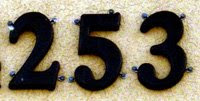253 is a star number. The nth star number is given by the formula 6n(n - 1) + 1.NGC 253, also known as the Silver Coin Galaxy or the Sculptor Galaxy, is a large spiral galaxy in the constellation Sculptor.

Source: Wikipedia

## Monday, April 27, 2009

### 94

94 = 2 x 47.

94 is the smallest even number greater than 4 that cannot be written as a sum of two twin primes.

Add the prime factors of 94 and the result is 94 reversed: 2 + 47 = 49.

94 equals the sum 2 + 3 + 4 + . . . + 16 minus the sum of primes less than 16.94 is the atomic number of the radioactive element plutonium.

Source: Prime Curios!

## Friday, April 24, 2009

### 389

389 is a prime number.

389 is the first in the smallest set of four consecutive primes (389, 397, 401, 409) that are congruent to 1 (mod 4). In other words, dividing each of these primes by 4 leaves a remainder of 1.

389 has a representation as a sum of two squares: 389 = 102 + 172.

389 is the hypotenuse of a primitive Pythagorean triple: 3892 = 1892 + 3402.St. Patrick was born in Roman Britain in the year
389 A.D.

Source:
Prime Curios!

## Thursday, April 23, 2009

### 509

509 is a prime number. Because 2 x 509 + 1 = 1019 is a prime, 509 is also a Sophie Germain prime.

509 is the sum of three consecutive squares: 122 + 132 + 142 = 509.

In base 4, 509 is the palindrome 13331.

509 has a representation as a sum of two squares: 509 = 52 + 222.

509 is the hypotenuse of a primitive Pythagorean triple: 5092 = 2202 + 4592.In the Clint Eastwood movie Firefox, 509 is the number on the conning tower of the submarine beneath the icepack.

Source: Prime Curios!

## Wednesday, April 22, 2009

### 903

903 = 3 x 7 x 43.

903 is the 42nd triangular number (the sum of all integers from 1 to 42).

To get the largest prime number of 903 decimal digits, subtract 903 from 10903.

903 is a number that cannot be written as a sum of three squares.903 is a telephone area code in the state of Texas (Texarkana region).

## Tuesday, April 21, 2009

### 1415

1415 = 5 x 283.

1415 is a number that cannot be written as a sum of three squares.

1415 is the sum of nine consecutive primes: 1415 = 137 + 139 + 149 + 151 + 157 + 163 + 167 + 173 + 179.

1415 is a centered icosahedral number.In the Battle of Agincourt on Oct. 25,
1415, the archers of Henry V of England were instrumental in defeating an army of French knights.

Source:

## Monday, April 20, 2009

### 717

717 = 3 x 239.

717 is also a palindrome in base 2: 1011001101.

717 is the largest three-digit palindrome such that if it is raised to the sum of its digits plus the sum of its digits plus one, the result is a prime number: 717(7+1+7) + (7 + 1 + 7) + 1.

The sum of the squares of the 717 consecutive primes starting from 1847 and 6959 is in both cases palindromic: 18708380781 and 77817671877.The Boeing 717 was a twin-engine, single-aisle jet airliner for about 100 passengers.Source: Prime Curios!

## Friday, April 17, 2009

### 306

306 = 2 x 32 x 17.

3*
306 + 0*306 + 6*306 - 1 is a prime number.

306 is the number of five-digit triangular numbers.

306 is the sum of four consecutive primes: 71 + 73 + 79 + 83 = 306.

306 has a representation as a sum of two squares: 306 = 92 + 152.Source:
Prime Curios!

## Thursday, April 16, 2009

### 212

212 = 22 x 53.

212 has a representation as a sum of two squares: 212 = 42 + 142.

212 is the smallest palindromic number with sum of digits prime (5) and number of prime digits prime (2).

15 circles divide a plane into at most
212 regions.The boiling point of water under standard conditions is
212 degrees on the Fahrenheit scale.

Source:
Number Gossip

## Wednesday, April 15, 2009

### 41

41 is a prime number.

41 is the smallest number such that the sum of its divisors equals three times its reverse: 41 + 1 = 3 x 14.

41 is the smallest prime such that the sum of factorials of its distinct digits is a square number: 4! + 1! = 52.

41 has a representation as a sum of two squares: 41 = 42 + 52.Accused murderess Lizzie Borden allegedly gave her father 41 whacks on Aug. 14, 1892, in Fall River, Mass.

Source: Prime Curios!

## Tuesday, April 14, 2009

### 14

14 = 2 x 7.

14 is the sum of the 2nd, 3rd, 4th, and 5th numbers: 2 + 3 + 4 + 5 = 14.

14 is the smallest number n such that n and 2n end with the same digit: 214 = 16,384.

14 is the largest number of regions into which four circles can divide a plane.The cuboctahedron is an Archimedean solid with
14 faces (eight triangles and six squares).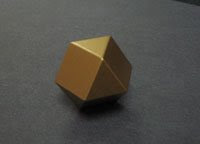Source:
Wikipedia

## Monday, April 13, 2009

### 413

413 = 7 x 59.

413 is a semiprime (a product of two primes), but (413 - 4)4 + (413 - 1)1 + (413 - 3)3 is prime with prime length, and contains a prime number of prime digits in its decimal expansion.

413 is a number n such that n and its reversal are both semiprimes: 314 = 2 x 157.

Inserting 9 between adjacent digits of 413 makes it a prime: 49193.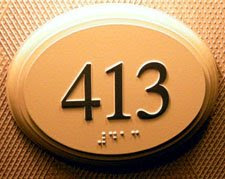Telephone area code 413 covers Springfield, Mass.

Source: Prime Curios!

## Friday, April 10, 2009

### 614

614 = 2 x 307.

614 is the smallest number that can be written as the sum of three squares in nine ways: 614 = 12 + 172 + 182 = 22 + 92 + 232 = 22 + 132 + 212 = 32 + 112 + 222 = 62 + 72 + 232 = 62 + 172 + 172 = 72 + 92 + 222 = 102 + 152 + 172 = 112 + 132 + 182.

614 is 21212 in base 4.

614 is the sum of six positive fifth powers: 614 = 35 + 35 + 25 + 25 + 25 + 25.Telephone area code 614 covers Columbus, Ohio.

## Thursday, April 9, 2009

### 2905

2905 = 5 x 7 x 83.

2905 is a number of the form n(2n + 13), for n = 35.

2905 is the product of the 23rd prime number and the 23rd composite number: 2905 = 83 x 35.NGC-2905 is a galaxy in the constellation Leo.

## Wednesday, April 8, 2009

### 397

397 is a prime number.

397 is conjectured to be the largest prime that can be represented uniquely as the sum of three positive squares (32 + 82 + 182).

397 is a Cuban prime. Cuban primes, named after differences between successive cubic numbers, have the form n3 - (n - 13).

397 has a representation as a sum of two squares: 397 = 62 + 192.

397 is the hypotenuse of a primitive Pythagorean triple: 3972 = 2282 + 3252.397 Vienna is an asteroid discovered in 1894.

## Tuesday, April 7, 2009

### 138

138 = 2 x 3 x 23.

138 is the smallest possible product of 3 primes, one of which is the concatenation of the other two.

138 is a value of n for which n!!! - 1 is prime.

12 × 483 = 5,796 = 42 × 138.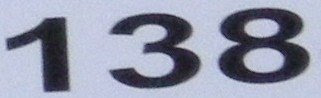NGC-138 is a spiral galaxy in the constellation Pisces.

Source: Number Gossip

## Monday, April 6, 2009

### 38

38 = 2 x 19.

38 is the largest even number that cannot be written as the sum of two odd composite numbers.

38 is the magic constant in the only possible magic hexagon (which uses all the integers up to and including 19).

38 is the number of integer partitions of 17 into distinct parts.The snub cube is an Archimedean solid with 38 faces.## Friday, April 3, 2009

### 270

270 = 2 x 33 x 5.

270 is a number n such that n2 + 1 is prime: 2702 + 1 = 72901, a prime.

270 is the smallest number whose sum of divisors (720) equals 10 times its reverse.

10! has
270 divisors.

270 is a number n such that 6n - 1 and 6n + 1 are twin primes: 6*270 - 1 = 1619 and 6*270 + 1 = 1621.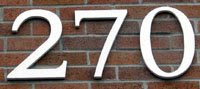270 is one the standard numerical values for resistors.

Source:
Number Gossip

## Thursday, April 2, 2009

### 89

89 is a prime number.

89 is the smallest prime whose digits, 8 and 9, are composites.

89 is the eleventh Fibonacci number.

89 is the only two-digit number that is the sum of its first digit and its second digit squared: 8 + 92 = 89.A sunflower head often displays florets in spirals of 55 and 89 around the outside.

Source: Number Gossip

## Wednesday, April 1, 2009

### 557

557 is a prime number.

557 is a Chen prime. A prime number p is called a Chen prime if p + 2 is either a prime or a product of two primes: (557 + 2 = 559 = 13 x 43). Chen primes are named after Jing Run Chen, who proved in 1966 that there are infinitely many such primes.

557 has a representation as a sum of two squares: 557 = 142 + 192.

557 is the hypotenuse of a primitive Pythagorean triple: 5572 = 1652 + 5322.USS Johnston (DD-557) was a World War II destroyer in the service of the U.S. Navy.

Source: Wikipedia